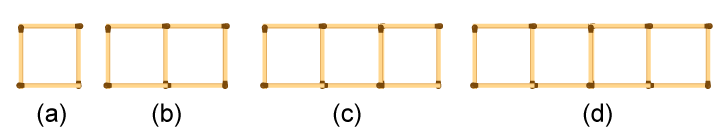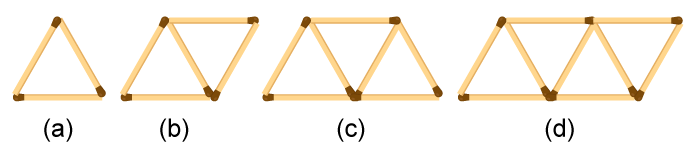# (a) Look at the following matchstick pattern of squares (Fig ure. The squares are not separate. Two neighbouring squares have a common matchstick. Observe the patterns and find the rule that gives the number of matchsticks (b) Figure gives a matchstick pattern of triangles. As in Exercise 11 (a) above, find the general rule that gives the number of matchsticks in terms of the number of triangles.Solution:

(a) From the figure we observe that

The matchstick pattern, the number of matchsticks are 4, 7, 10 and 13, which is 1 more than the thrice of the number of squares in the pattern

Therefore, the pattern formed here is 3x + 1, where x is the number of squares

(b) From the figure we observe that

The given matchstick pattern, the number of matchsticks are 3, 5, 7 and 9 which is 1 more than the twice of the number of triangles in the pattern.

Therefore, the pattern formed here is is 2x + 1, where x is the number of triangles.(0)(0)